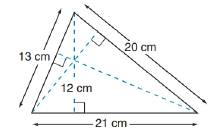Chapter 8.1, Problem 48E### Elementary Geometry for College St...

6th Edition
Daniel C. Alexander + 1 other
ISBN: 9781285195698

#### Solutions

Chapter
Section### Elementary Geometry for College St...

6th Edition
Daniel C. Alexander + 1 other
ISBN: 9781285195698
Textbook Problem
1 views

# In Exercises 47 to 50, use the fact that the area of the polygon is unique.In the triangle whose sides are 13, 20, and 21 cm long, the length of the altitude drawn to the 21-cm side is 12 cm. Find the lengths of the remaining altitudes of the triangle.To determine

To find:

The lengths of the altitudes of the triangle.

Explanation

Converting theoretical statement into mathematical statement, identifying the geometric figure and the calculation is done by suitable geometric formulae.

Calculation:

Given,

In a triangle ABC,

The length of the sides are

AB=13 cm

BC=21 cm

AC=20 cm

The length of the altitude h1=12 cm

To find the length of the altitude h2 and h3.

Area of the triangle ΔBCA(A):

Let BC is the base of the triangle ABC and AD is the height of the triangle ABC.

Here, BC=b1=21 cm

Area of the triangle A=12b1h1

=12(21)(12)

=12(252)

Area of the triangle is A=126 cm2

Find h2:

For the triangle ΔCAB

Let CA is the base of the triangle ΔCAB, and h2 is the height of the triangle.

Here, CA=b2=20 cm and BE=h2

Thus, the area of the triangle (A)=12b2h2

126=12(20)h2

126=10h2

126=10h2

Divide by 10 on both sides,

12610=10h210

12

### Still sussing out bartleby?

Check out a sample textbook solution.

See a sample solution

#### The Solution to Your Study Problems

Bartleby provides explanations to thousands of textbook problems written by our experts, many with advanced degrees!

Get Started

#### Which is the best graph of r = 1 − sin θ for 0 ≤ θ ≤ π?

Study Guide for Stewart's Single Variable Calculus: Early Transcendentals, 8th

#### True or False:

Study Guide for Stewart's Multivariable Calculus, 8th

#### Simplify each expression. 323/5

College Algebra (MindTap Course List)